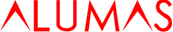ABOUT US catalog
Determination of AL Profiles

Example

1. BP-8080 secondary moment , I = 137.21*10^4mm^4
2. If loaded with 500kg , to draw point (1) on line W.
3. Supporting distance L=1000 m/m , to draw point (2) on line L.
4. Draw a horizontal line from point (1),draw a vertical line form point (2) to cross at point (3).
5. BP-8080, the point of secondary moment I is point(4), draw horizontal line from point (4),draw an oblique parallel line from point (3) to cross at point (5).
6. Draw a horizontal line from (5) to know the distortions.

Remark

1. Steps sequence can be changed according to the situation requirement.
2. The distortion caused by material self weight may reduce the calculation to a half value.
3. If the precision value is required please calculate as following.
4. The formulas is calculated the base of the distortions of profile supporting distance is L= 1/1000 m/m.No.147, Sec. 1, Jingguo Rd., East Dist., Hsinchu City 300, Taiwan (R.O.C.)

TEL：+886-3-5429988　FAX：+886-3-5319266

www.alumas.com.twCopyright © 2019 ALUMAS All rights reserved . Design by PROC